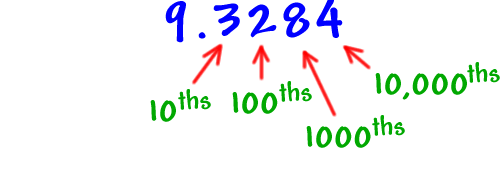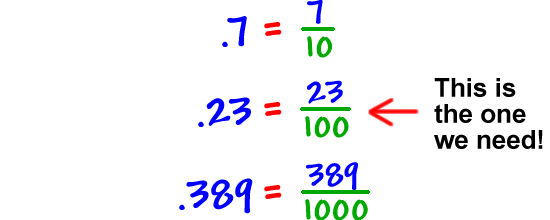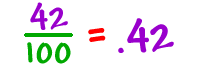First, I need to remind you about something with decimals:

Remember place values?So...At first, we'll do this in two steps:

 1
Use the word "percent" to convert the percent to a fraction.
 2
Use decimal place values to convert the fraction to a decimal.

Let's go!

Convert 42% to a decimal:

 Step 1:Don't simplify the fraction! Step 2: